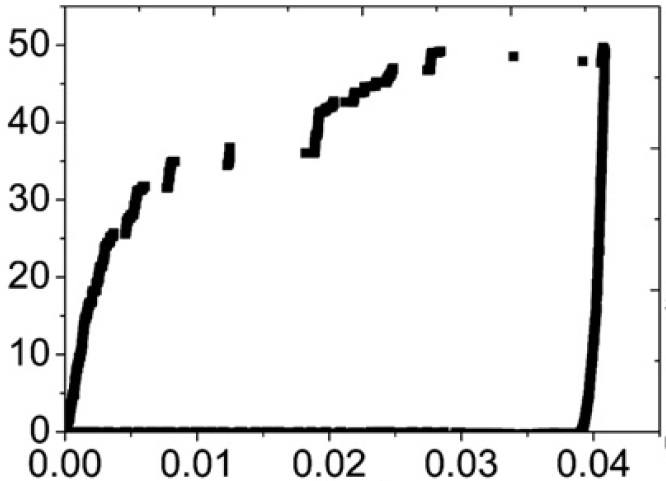# How to extract data from existing JPEG/TiFF graph?

• MATLAB
Gold Member
Is there any method to extract data from a graph in JPEG/TIFF format, using simple software such as MATLAB? I have a graph as shown below:I only have this picture but no raw data and I want to plot the black curve in this graph. How to get approximate numerical data of the x and y axis? Thank you.

#### Attachments

DrClaude
Mentor
I don't know how to do it simply in Matlab. For such tasks, I use DataThief.

•kelvin490
You can use getframe()

Code:
plot(1:10);
structFrame = getframe(); % Get structure
rgbImage = structFrame.cdata;
figure;
imshow(rgbImage);